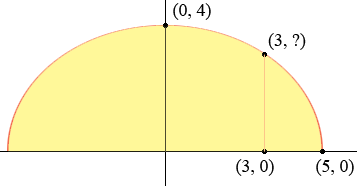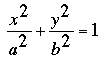SEARCH HOMEMath Central Quandaries & QueriesQuestion from jessica, a student: An arch in the form of a semi ellipse has a span of 10 meters and a central height of 4 m. Find the heights of the arch at a point of 3 meters from the semi minor axis.Hi Jessica,

Assume the ellipse is in standard position with its centre at the origin, the major axis along the x-axis and its minor axis along the y-axis.The equation of the ellipse is thenSince (5, 0) and (0, 4) are on the ellipse if you substitute these values into the equation you can solve for a and b. Then substitute x = 3 into the equation and solve for y.

PennyMath Central is supported by the University of Regina and The Pacific Institute for the Mathematical Sciences.